# Solving First-Order Initial Value Problems

Problem

You need to numerically solve a first-order differential equation of the form: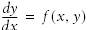y(0) = a

Solution

This is a standard initial value problem and you can implement any of a number of standard numerical integration techniques to solve it using Excel and VBA.

Discussion

Consider a specific initial value problem such as: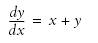y(0) = a

The exact solution for this problem is:

y = ex - x - 1

Figure 11-1 shows a plot of this exact solution.

Normally, if you can find an exact solution, you wouldn't resort to numerical integration. However, I chose this problem so that you can compare the numerical results with the exact solution.

In this recipe, I will show you how to implement Euler's method . This isn't necessarily the best method, since it requires small step sizes to ensure accuracy. However, it's simple enough to show you the mechanics of implementing such a method in Excel and VBA without clouding the issue. In Recipe 11.2, I'll show you how to implement a much better method. In Recipe 11.3, I'll show you why Euler's method may not be the best choice for some problems.

Euler's method is based on taking the first two terms of a Taylor series expansion of a function to predict the value of the function at some point, knowing the value of the function at some other point plus some information about the derivatives of that function. In this case, you can write the Taylor series expansion of y as follows: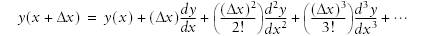Euler's method considers only the first two terms on the right side of this equation, ignoring all higher-order terms, which collectively constitute the truncation error for this method. For the problem we're considering here, we know dy/dxit's the given differential equationtherefore, we can use the first two terms in the Taylor series expansion of y to determine y at successive increments along x, starting at the given initial value. This scheme is very easy to implement in Excel and VBA. In fact, you can implement this scheme easily in a standard spreadsheet without the use of VBA, or you can write a VBA subroutine, which I think is a more versatile approach. I'll show you how to do both.

Figure 11-1. Exact solution of an example initial value problem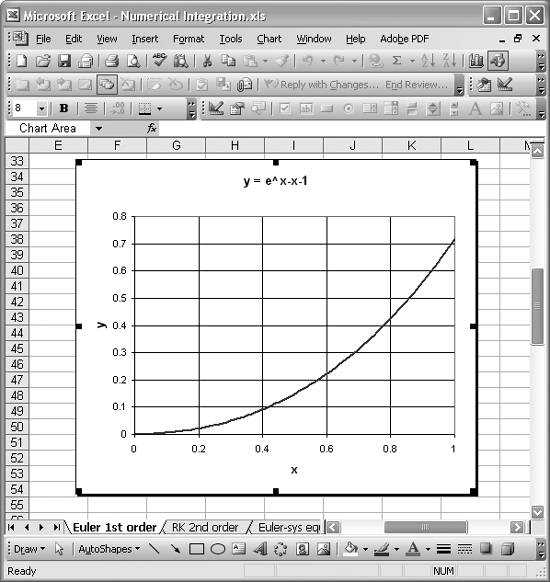Figure 11-2 shows a spreadsheet I set up implementing Euler's method using only spreadsheet formulas.

The three separate tables you see in Figure 11-2 are solutions to the same problem using three different step sizes, dx (labeled dx in Figure 11-2). You need only one table to solve the problem, but I include three to show the differences in results using different step sizes.

The tables are fairly straightforward. The columns labeled x contain formulas like =M5+\$N\$1, which simply compute a new x-value given the previous x-value plus the step size. The columns labeled y contain Euler's formula for predicting the next y-value. The spreadsheet formula looks like =N5+(M6-M5)*(M5+N5). The (M6-M5) part of this formula is the step size, which could have been simply \$N\$1 as well. The (M5+N5) part is the derivative of y from the given differential equation.

Figure 11-2. Euler's method using only spreadsheet formulasThe columns labeled y exact contain formulas like =EXP(M6)-M6-1, corresponding to the equation for the exact solution discussed earlier. And finally, the error columns contain formulas like =(O6-N6), which is just the difference between the exact solution and the approximate solution.

The error decreases as the step size decreases, as you would expect. Note, however, that you have to perform far more computations with the smaller step size to cover an equivalent range of x-values. For example, if you wanted to compute the solution over the range x = [0,1], you'd need 10 rows of calculations using a step size of 0.1, 100 rows using a step size of 0.01, and 1,000 rows using a step size of 0.001. Clearly, if you require a small step size and want to cover a reasonable range of x-values for a given problem, your spreadsheet tables will grow very large. This becomes cumbersome, especially when you want to plot the results, change the range, or change your step size. VBA offers a better approach.

Using VBA

Example 11-1 shows the VBA subroutine I wrote implementing Euler's method for our example problem.

Example 11-1. DoEuler1stOrder

 ```Public Sub DoEuler1stOrder( ) Dim yn As Double Dim yn1 As Double Dim xn As Double Dim dx As Double Dim n As Integer   yn = 0 xn = 0 dx = 0.001 n = 1000   For i = 1 To n yn1 = yn + (xn + yn) * dx xn = xn + dx yn = yn1 ActiveSheet.Cells(i + 1, 1) = xn ActiveSheet.Cells(i + 1, 2) = yn Next i End Sub ```

This subroutine computes estimated values for y over a range of x-values and places the results in the active spreadsheet starting at row 2 in columns A and B.

The variables declared at the beginning of this subroutine are used to store yx (yn), yx+1 (yn1), x (xn), and dx (dx). n is a counter variable representing the number of steps to take.

The For loop iterates from 1 to n, computing estimates for y at each step. The line yn1 = yn + (xn + yn) * dx computes the predicted y-value given the previous y-value, the step size, and the derivative of y from the original differential equation. After this calculation and after updating xn and yn, the subroutine writes the results to the active spreadsheet. The line ActiveSheet.Cells(i + 1, 1) = xn writes the x-value to row i+1 and column 1 in the R1C1 cell reference style (column 1 corresponds to column A). The line ActiveSheet.Cells(i + 1, 2) = yn writes the y-value to row i+1, column 2.

I set up a button on a spreadsheet (just like the one I showed in Recipe 9.3) to call this subroutine. When I press the button, the spreadsheet is populated with the computed results. While this approach works fine, it suffers one of the problems mentioned earlier with using pure spreadsheet formulas: when using a small step size while covering a reasonable range of x-values, the spreadsheet contains a large number of data points. The subroutine shown in Example 11-1 would fill 1,000 rows of data, which could become cumbersome, as discussed earlier.

To mitigate this problem, you can readily add a few lines of code to DoEuler1stOrder that will output results every 100 steps or so in order to keep the number of output rows manageable.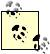This is a common technique in numerical and simulation work. For example, real time simulations and video games that use physics-based effects use numerical integration to solve equations of motion. These algorithms typically use very small time steps that would render the simulation or game extremely slow if the graphics were updated every time step. Instead, the underlying computations are computed at small steps but the graphics are updated at a rate that keeps the simulation or game at a real-time pace.

Example 11-2 shows the modified subroutine using this controlled output technique.

Example 11-2. Modified DoEuler1stOrder

 ```Public Sub DoEuler1stOrder( ) Dim yn As Double Dim yn1 As Double Dim xn As Double Dim dx As Double Dim n As Integer Dim c As Integer Dim k As Integer   yn = 0 xn = 0 dx = 0.001 n = 1100 c = n / 10 k = 1   For i = 1 To n yn1 = yn + (xn + yn) * dx xn = xn + dx yn = yn1   If c >= (n / 10) Then ActiveSheet.Cells(k + 1, 1) = xn ActiveSheet.Cells(k + 1, 2) = yn k = k + 1 c = 0 Else c = c + 1 End If Next i End Sub ```

I added two new variables, c and k. c is a counter variable used to determine when to output results to the spreadsheet, and k is a counter variable used to track the output row.

I decide to output only 10 rows of data while keeping the step size at 0.001. I still wanted to cover a range of x-values from 0 to 1, which meant I had to make n equal to 1,100. Thus, output will be delivered to the spreadsheet every n/10 steps, that is, whenever c equals n/10.

The results of this new subroutine are shown in Figure 11-3.

Figure 11-3. Results using VBA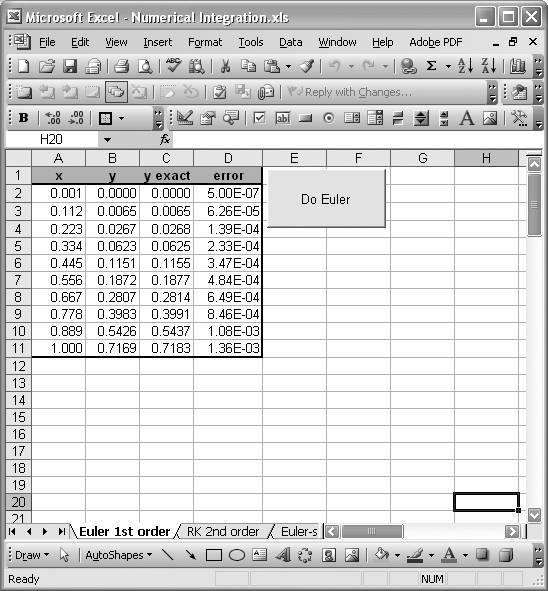Here you can see the little table I set up to receive the 10 rows of results. You can also see the button I set up to run the subroutine. This version is much more manageable and gives you greater flexibility when you need to modify your solution algorithm. For example, to change the step size to 0.0001 while keeping the results table the same size, all you need to do is change dx to 0.0001 and n to 11,000 in DoEuler1stOrder and then rerun it.

If you try Euler's method for a problem and see that it gives poor results, you might then decide to implement a higher-order method such as the Runge-Kutta method. This latter method would be cumbersome to set up in a spreadsheet alone; however, if you're already using VBA, you can modify your solution subroutine without too much effort and then recompute the results.

Check out Recipe 11.2 to see how to extend the VBA techniques from this recipe to handle second-order equations.Excel Scientific and Engineering Cookbook (Cookbooks (OReilly))
ISBN: 0596008791
EAN: 2147483647
Year: N/A
Pages: 206
Authors: David M Bourg